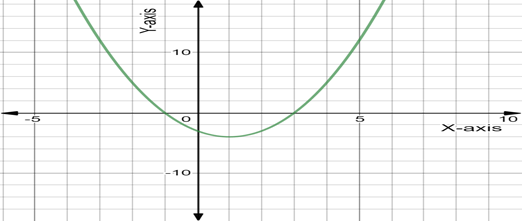# The blank in the statement “the solution of the equation x 2 − 2 x − 3 = 0 are the intercepts of the graph y = x 2 − 2 x − 3 ”.### Precalculus: Mathematics for Calcu...

6th Edition
Stewart + 5 others
Publisher: Cengage Learning
ISBN: 9780840068071### Precalculus: Mathematics for Calcu...

6th Edition
Stewart + 5 others
Publisher: Cengage Learning
ISBN: 9780840068071

#### Solutions

Chapter 1.9, Problem 1E
To determine

## To fill: The blank in the statement “the solution of the equation x2−2x−3=0 are the         intercepts of the graph y=x2−2x−3 ”.

Expert Solution

The complete statement is the solution of the equation x22x3=0 are the xintercepts of the graph y=x22x3 .

### Explanation of Solution

Given information:

The solution of the statement x22x3=0 are the intercepts of the graph y=x22x3 .

Consider the provided statement “ x22x3 are the intercepts of the graph y=x22x3 .” x -intercepts is a point where a line crosses the x- axis.

y intercepts is a point where a line crosses the y axis.

So, the equation is x22x3=0 , we check the equation for y=0 .

Here y=0 then the equation becomes:

x22x3=0x22xx3=0x(x2)+1(x2)=0(x+1)(x2)=0

Either x+1=0 or x2=0 :

Simplified further as x=1 or x=2 .

Substitute the value of x in the equation x22x3=y .

Now for x=1 substitute the value of x in a given equation y=x22x3 .

y=x22x3y=12213y=123y=4

Now for x=2 substitute the value of x in the given equation y=x22x3 .

y=x22x3y=22223y=443y=3

The graph of the equation is open upward.Here the graph of line crosses the x -axis ,so the graph statement x22x3=0 has x intercepts, because there is no line of intersection at y -axis.

Thus, the complete statement, “the solution of the statement x22x3=0 are the x intercepts of the graph y=x22x3 ”.

### Have a homework question?

Subscribe to bartleby learn! Ask subject matter experts 30 homework questions each month. Plus, you’ll have access to millions of step-by-step textbook answers!